Courses

# Strength of Materials - 3

## 20 Questions MCQ Test Mock Test Series for SSC JE Mechanical Engineering | Strength of Materials - 3

Description
This mock test of Strength of Materials - 3 for SSC helps you for every SSC entrance exam. This contains 20 Multiple Choice Questions for SSC Strength of Materials - 3 (mcq) to study with solutions a complete question bank. The solved questions answers in this Strength of Materials - 3 quiz give you a good mix of easy questions and tough questions. SSC students definitely take this Strength of Materials - 3 exercise for a better result in the exam. You can find other Strength of Materials - 3 extra questions, long questions & short questions for SSC on EduRev as well by searching above.
QUESTION: 1

### The resistance per unit area, offered by a body against deformation is known as:

Solution:
• The force of resistance per unit area, offered by a body against deformation is known as stress.
• The external force acting on the body is called the load or force.
• When a body is subjected to some external force, there is some change of dimension of the body. The ratio of change of dimension of the body to the original dimension is know as strain.
QUESTION: 2

### Which of the following load does not act on the considerable length of the beam?

Solution:

Point load is that load which acts over a small distance. Because of concentration over small distance this load can may be considered as acting on a point. Point load is denoted by P and symbol of point load is arrow heading downward (↓).

Uniformly distributed load is that whose magnitude remains uniform throughout the length.

Uniformly varying load is further divided into two types:

• Triangular Load: whose magnitude is zero at one end of span and increases constantly till the 2nd end of the span.
• Trapezoidal Load: which is acting on the span length in the form of trapezoid. Trapezoid is generally form with the combination of uniformly distributed load (UDL) and triangular load.
QUESTION: 3

### The shear stress on a beam section is maximum:-

Solution:

Shear stress on a beam section is:

(i) Zero at extreme fibres

(ii) Maximum at neutral axis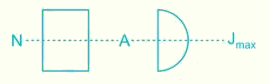QUESTION: 4

Maximum Shear strain Energy theory was postulated by:

Solution:

. Maximum Principal stress theory was postulated by Rankine. It is suitable for brittle materials.

2. Maximum Principal strain theory was postulated by St Venant. This theory is not accurate for brittle and ductile materials both.

3. Maximum Shear stress theory was postulated by Tresca. This theory is suitable for ductile materials. Its results are the safest.

4. Maximum shear strain energy theory was postulated by Von-mises. Its results in case of pure shear are the accurate for ductile materials.

QUESTION: 5

The maximum deflection due to a load W at the free end of a cantilever of length L and having flexural rigidity EI is

Solution: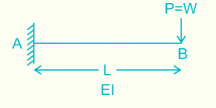The maximum deflection will occur at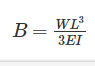QUESTION: 6

If section modulus of a beam is increased, the bending stress in the beam will:

Solution:

Bending Moment Equation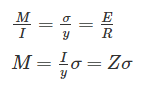For the constant bending moment: σ ∝ 1/Z

So, bending stress is inversely proportional to the Section Modulus 'YH

QUESTION: 7

When the shear force diagram is a parabolic curve between two points, it indicates that there is a

Solution:

The general relationship between the shear force diagram, bending moment diagram and loading diagram will be:

2. Bending moment diagram is 1higher than shear force diagram.

3. For the uniformly varying load on the beam, the shear force diagram is parabolic in nature.

4. For the uniformly varying load on the beam, the bending moment diagram is also parabolic but is 1o higher than shear force diagram.

QUESTION: 8

Mohr’s circle can be used to determine the following stress on an inclined surface:

A. Principal stress

B. Normal stress

C. Tangential stress

D. Maximum shear stress

Solution:

Mohr circle is a graphical representation of plane stress which helps in determining the relationships between normal and shear stresses acting on any inclined plane at a point in a stressed body.

Mohr’s circle of stresses is a graphical method of finding normal, tangential and resultant stresses on an oblique plane.

QUESTION: 9

The ratio of moment of inertia of circular plate to that of square plate of equal depth is:

Solution: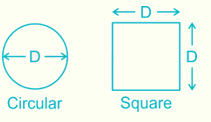Moment of inertia (I1) of circular plate of diameter “D” is given by:

.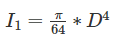Moment of inertia (I2) of square plate of side equal to diameter “D” of circular plate is given by: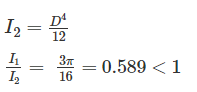QUESTION: 10

Which material has highest value of poison’s ratio?

Solution:

Elastic Rubber: 0.5

Wood: Because wood is orthotropic, 12 constants are required to describe elastic behaviour: 3 moduli of elasticity, 3 moduli of rigidity, and 6 Poisson’s ratios (vary from 0.02 to 0.47). These elastic constants vary within and among species and with moisture content and specific gravity.

Copper: 0.33

Steel: 0.27 - 0.30

QUESTION: 11

The major and minor principal stresses at a point are 3Mpa and -3Mpa respectively. The maximum shear stress at the point is

Solution:

Maximum shear stress (τ) is given by: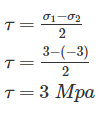QUESTION: 12

The equivalent length of a column of length L having one end fixed and the other end free is

Solution:

Effective length of columns under different end conditions are:

1. For both ends hinged: Le = L

2. One end fixed and another end free: Le = 2L

3. Both ends fixed = Le=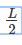4. One end fixed and other is hinges: Le=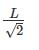QUESTION: 13

The radius of Mohr’s circle for two equal and unlike principal stresses of magnitude “p” is:

Solution:

Radius of Mohr’s circle is given by:

r=

At principal plane, σx = σ1, σy = σ2

τxy = 0

r=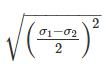σ= p , σ2 = -p

r=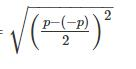r = p

QUESTION: 14

A rectangular beam is 24 cm wide and 50 cm deep, its section modulus is given by:

Solution:

Section modulus is defined as the ratio of moment of inertia of a beam about its C.G to the maximum distance of extreme x-section of the beam (Ymax).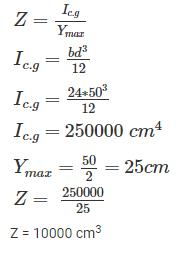QUESTION: 15

The stress at a point in a bar is 200 MPa tensile. Determine the intensity of maximum shear stress in the material.

Solution: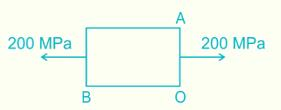Stress on x face, A(200, 0) and Stress on y face, B(0, 0)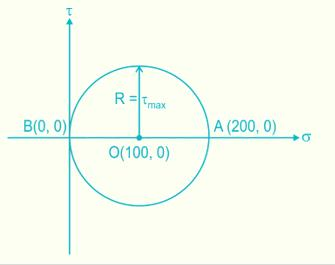Maximum shear stress = Radius of Mohr circle

τmax= σ/2 = 100 MPa

QUESTION: 16

The correct relation between Modulus of elasticity (E), Shear Modulus (G) and Bulk modulus (K) is:

Solution:

Relation between Modulus of elasticity (E), Shear Modulus (G) and Bulk modulus (K) is given by: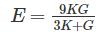Now, rewriting the equation as follows:

3KE + GE = 9KG

3KE – 9KG = - GE

K(3E – 9G) = - GE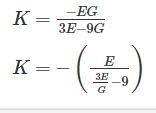QUESTION: 17

The total area under the stress-strain curve of a mild steel specimen tested up to failure under tension is a measure of its:

Solution:

Strength is defined as the ability of the material to resist, without rupture, external forces causing various types of stresses. Breaking strength is the ability of a material to withstand a pulling or tensile force.

Toughness is defined as the ability of the material to absorb energy before fracture takes place. In other words, toughness is the energy for failure by fracture. Toughness is measured by a quantity called modulus of toughness. Modulus of toughness is the total area under a stress-strain curve in tension test, which also represents the work done to fracture the specimen.

Hardness is defined as the resistance of a material to penetration or permanent deformation. It usually indicates resistance to abrasion, scratching, cutting or shaping.

Stiffness or rigidity is defined as the ability of the material to resist deformation under the action of external load. Modulus of elasticity is the measure of stiffness.

QUESTION: 18

What is the limit to Poisson's ratio?

Solution:

Limit of poisons ratio varies between -1 to 0.5.

μ = 0.5 for rubber.

QUESTION: 19

Slenderness ratio of a long column is

Solution:

Slenderness ratio ( λ ) of long column is defined as the ratio of Effective length of column to its least radius of gyration.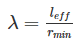QUESTION: 20

The neutral axis of a beam is subjected to _________ stress.

Solution:

The neutral axis is an axis in the cross-section of a beam (a member resisting bending) or shaft along which there are no longitudinal stresses or strains. If the section is symmetric, isotropic and is not curved before a bend occurs, then the neutral axis is at the geometric centroid. All fibers on one side of the neutral axis are in a state of tension, while those on the opposite side are in compression.